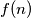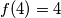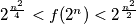### IMO Shortlist 1997 problem 24

Kvaliteta:
Avg: 0,0
Težina:
Avg: 0,0
For each positive integer$n$, let$f(n)$ denote the number of ways of representing$n$ as a sum of powers of 2 with nonnegative integer exponents. Representations which differ only in the ordering of their summands are considered to be the same. For instance,$f(4) = 4$, because the number 4 can be represented in the following four ways: 4; 2+2; 2+1+1; 1+1+1+1.

Prove that, for any integer$n \geq 3$ we have$2^{\frac {n^2}{4}} < f(2^n) < 2^{\frac {n^2}2}$.
Izvor: Međunarodna matematička olimpijada, shortlist 1997